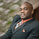2348 obejrzeń

(ii) Circle represent triggering of the trade
--------------------------------------------------------------------
1. Where to place stop loss?
2. Where to exit the trade?
4. How to apply money management rule?
5. How to trade gap opening?
```//  By SIMPLE TRADING TECHNIQUES

//   	Arrow represent trade setup
//   	Circle represent triggering of the trade
//--------------------------------------------------------------------

study(title="STT ENGULFING CANDLESTICK STRATEGY",overlay = true, shorttitle="STT ECS")
src = close, len = input(8, minval=1, title="Length")
up = rma(max(change(src), 0), len)
down = rma(-min(change(src), 0), len)
rsi = down == 0 ? 100 : up == 0 ? 0 : 100 - (100 / (1 + up / down))

//coloring method below

src1 = close, len1 = input(50, minval=1, title="UpLevel")
src2 = close, len2 = input(50, minval=1, title="DownLevel")
isup() => rsi > len1
isdown() => rsi < len2
barcolor(isup() ? green : isdown() ? red : na )

out = sma(close, 50)
data = rsi > len1 ? open > close ? close > open ? close >= open ? close >= open ? close - open > open - close ? high > out : na : na : na : na : na : na
data1 = rsi < len2 ? close > open ? open > close ? open >= close ? open >= close ? open - close > close - open ? low < out : na : na : na : na : na : na

plotchar(data1, char='↓', location=location.abovebar, color=red, text="ECS Sell")

tiggerlongcandle = (data == 1) and (high > high) ? 1 : 0
tiggershortcandle = (data1 == 1) and (low < low) ? 1 : 0
plotshape(tiggerlongcandle ? tiggerlongcandle : na, title="Triggered Long",style=shape.circle, location=location.belowbar, color=green, transp=0, offset=0, text="ECS")
plotshape(tiggershortcandle ? tiggershortcandle : na, title="Triggered Short",style=shape.circle, location=location.abovebar, color=red, transp=0, offset=0, text="ECS")

plot (out, color = black, linewidth = 3, title = "Trend - Long Term")
```

## Komentarzemcommorato
@mcommorato, Yes it's the 50-period simple moving average.
Odpowiedzshani_1694
i don't understand the question, can reword it please.
Odpowiedzshani_1694
this script is not for the pullback candlestick strategy, it is for another strategy that i am working on. in order the get the script for the pullback candlestick strategy search for it in the indicators public library.
Odpowiedzshani_1694
that is a trade setup (signal) but the trade was not trigger. ARROW represent trade setup while CIRCLE represent triggering of the trade
Odpowiedz